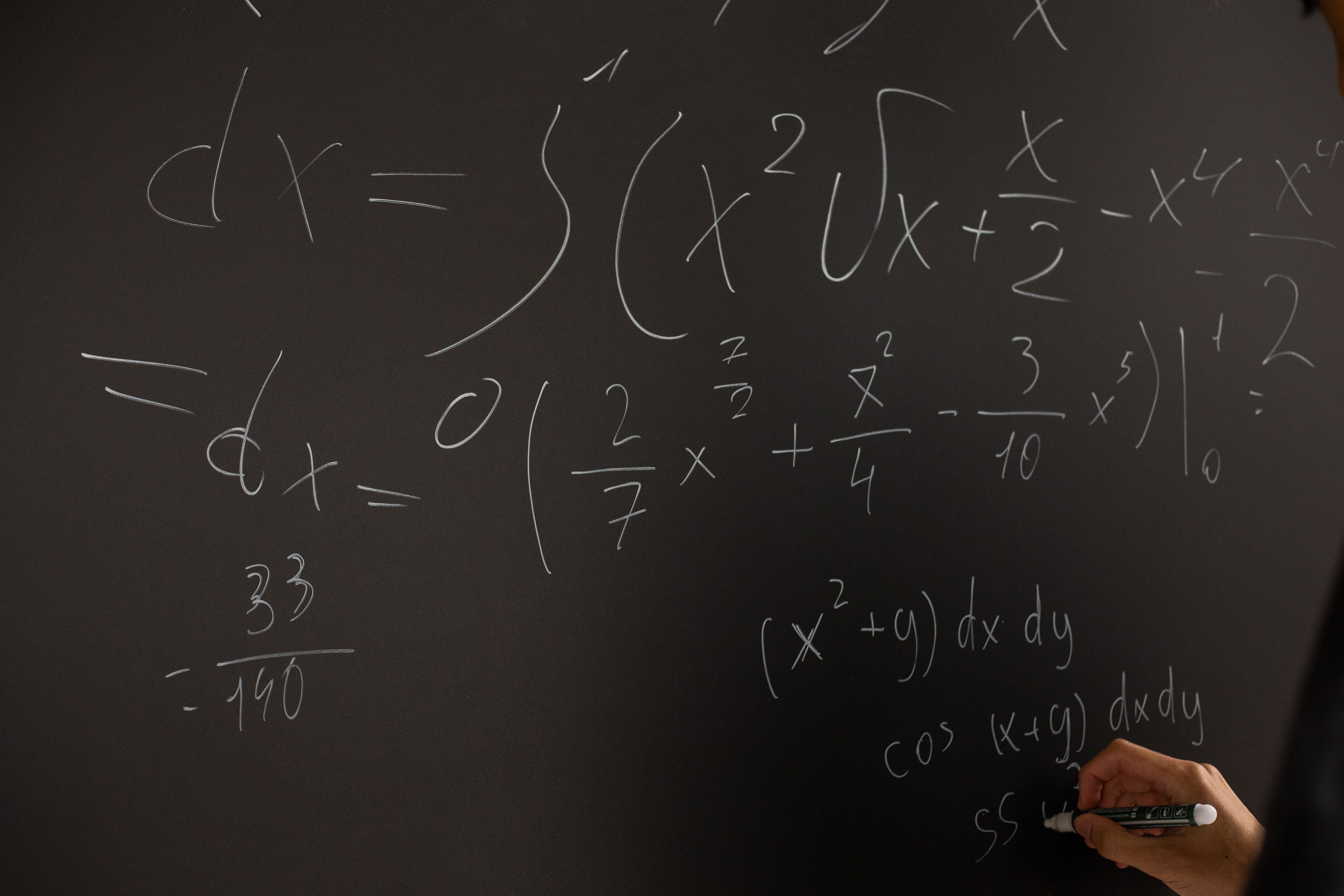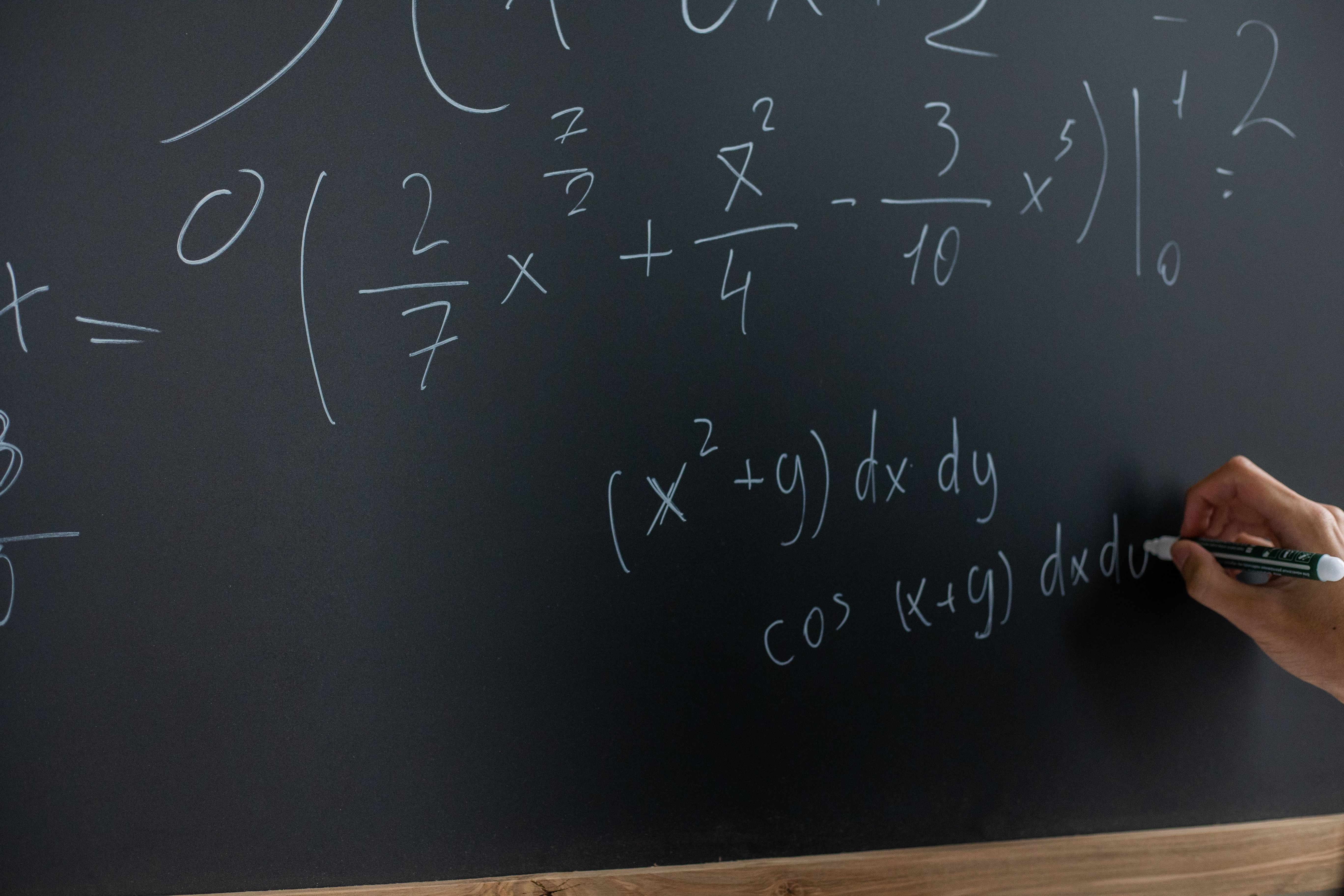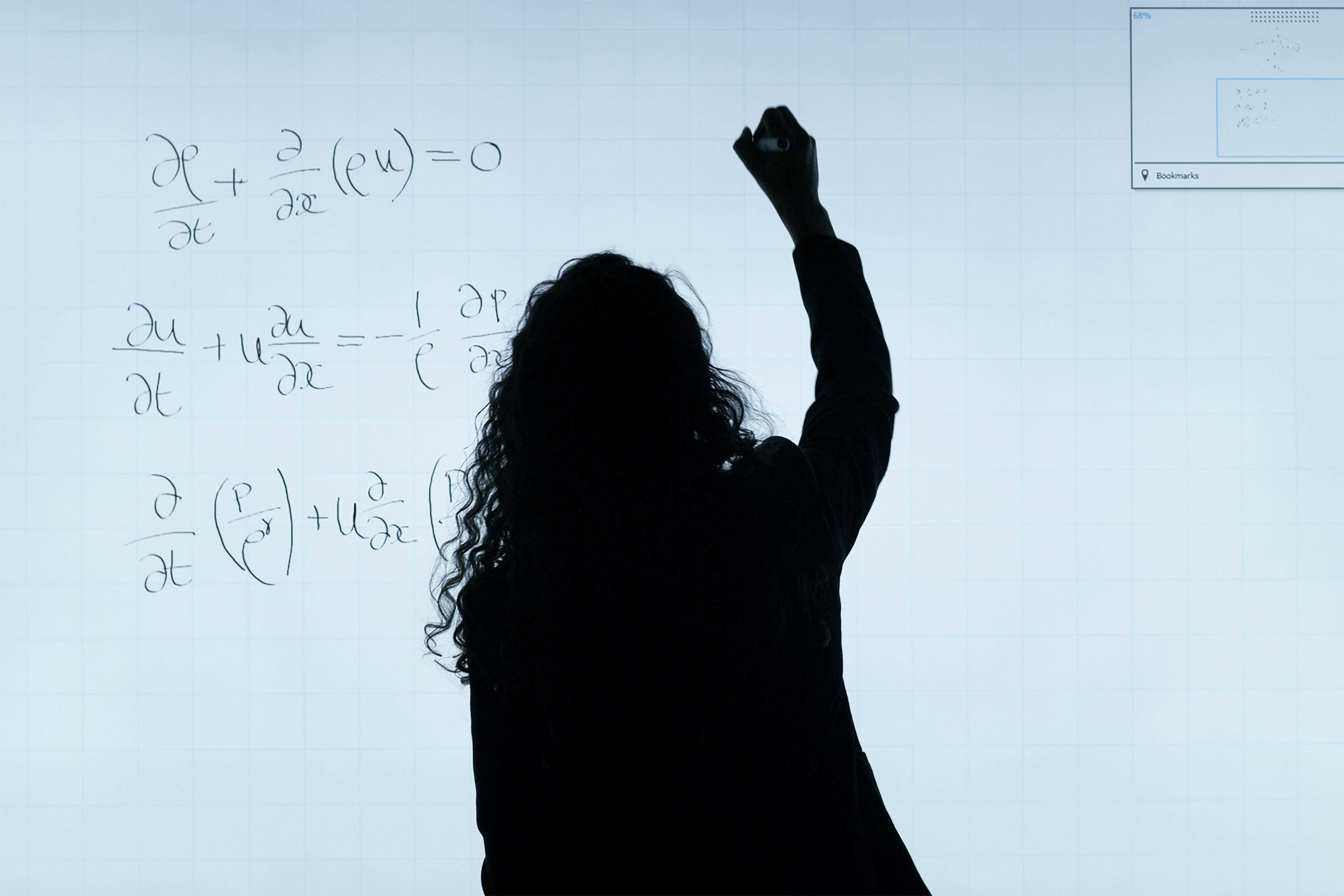# Can I take discrete math without calculus?

Can I Take Discrete Math without Calculus?

# Can I Take Discrete Math without Calculus?Discrete math is an important course for computer science, mathematics and engineering students. It deals with mathematical structures that are countable and distinct, like graphs and networks. One of the common questions students have is whether they can take discrete math without calculus. In this article, we will explore this question and provide answers to some of the most frequently asked questions about discrete math.

## Can I Take Discrete Math without Calculus?

The answer is yes, you can take discrete math without calculus. Calculus isn’t really needed to understand discrete math. However, some universities may require calculus as a prerequisite for the course. Thus, it’s important to check with your department and university to know the prerequisites for the course.Discrete math is a different type of math compared to calculus. Calculus deals with continuous functions, while discrete math deals with distinct objects. The concepts in discrete math are different from calculus and don’t require calculus knowledge to understand it. Moreover, if calculus is a prerequisite for the class, there are a number of good examples and homework problems that the professor might use that would indeed require calculus. Therefore, a solid foundation in calculus can be helpful but not necessary in understanding discrete math.

## FAQs

### What subjects do you need for discrete math?

Discrete math does not require any specific subject as a prerequisite. However, a solid foundation in algebra is helpful in understanding some of the concepts in discrete math. Other useful subjects include set theory, graph theory and probability. A good knowledge of logic is also important since discrete math deals with logical statements and proofs. Moreover, programming languages like Java and Python are useful in solving problems related to discrete math.

### What are the applications of discrete math?

Discrete math is a fundamental tool in computer science that forms the basis of many computer-related concepts. Applications of discrete math include algorithms, data structures, cryptography, coding theory, and artificial intelligence. Discrete math is used in solving problems related to computer networks, coding and decoding messages, routing, and much more. Discrete math is also used in finance and the stock market, as it helps in predicting trends and analyzing data.

### What are some good resources for learning discrete math?

There are many resources available to help you learn discrete math. Some of the best resources include textbooks like “Discrete Mathematics and Its Applications” by Kenneth H. Rosen or “Concrete Mathematics” by Ronald L. Graham, Donald E. Knuth, and Oren Patashnik. These books provide an easy to understand introduction to discrete math. Moreover, many free online courses are available on platforms like Coursera, edX, Udemy, and Khan Academy. They provide video lectures, quizzes, and assignments to help you learn the course. There are also many YouTube channels and blogs dedicated to teaching discrete math. In conclusion, there are many resources available on the internet and in libraries to help you learn discrete math.